### Create equations

To create an equation, insert an equation object in the document and then insert math elements in the equation.

tip:   When working with an equation, zoom in until it’s easy to read.

#### Create an inline equation

1)Click in text where you want to insert the equation. If the text is in a rotated text frame, unro­tate the frame first by selecting the rotated text frame and pressing Esc g 0 (zero).

2)Choose Special > Equations, or click the Equations button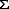at the right side of the document window.

3)On the Equations pod, choose one of the New Equation commands from the Equations pop-up menu. Small, Medium, and Large specify the font sizes used in the new equation.

A new equation object appears as a question mark in a frame anchored below the line with the insertion point. The first math element that you insert replaces the question mark prompt.

New inline equation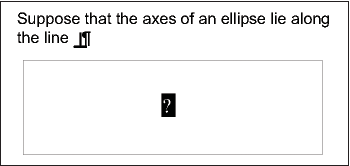4)Insert the math elements in the equation by typing the elements or by clicking items on the Equations pod.

Inserted elements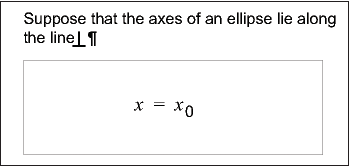5)Choose Shrink-Wrap Equation from the Equations pop-up menu. This option shrinks the frame around the equation and places the equation in the line of text at the anchor symbol.

Shrink-wrapped inline equation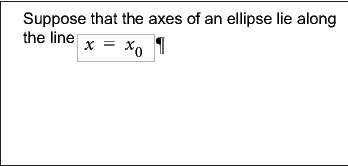6)If the equation seems too close to the text on either side, insert a space before or after the frame. FrameMaker treats the frame that contains an inline equation as a character and doesn’t provide extra space around it.

7)If the equation is too tall for the line, open up more space above or below the line. Use the Line Spacing pop-up menu on the formatting bar to turn off fixed line spacing.

#### Create an equation in a paragraph of its own (a display equation)

1)Click in an empty paragraph.

2)Apply a paragraph format from the Paragraph Catalog, or set up your own format. The para­graph format determines the alignment, vertical spacing, and any autonumber for the para­graph that contains the equation.

tip:   If you created the document from a book or report template, use the Equation paragraph format. You can also copy this format to your document, but you’ll probably want to make some changes (for example, to the Default Font and Spacing properties).

3)Click the Equations buttonat the right side of the document window.

4)On the Equations pod, choose one of the New Equation commands from the Equations pop-up menu. Small, Medium, and Large specify the font sizes used in the new equation.

A new equation object appears as a question mark in a frame anchored below the empty para­graph. The first math element you insert replaces the question mark prompt.

New equation in anchored frame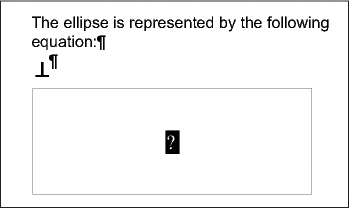5)Insert the math elements in the equation by typing the elements or by clicking items on the Equations pod.

Inserted elements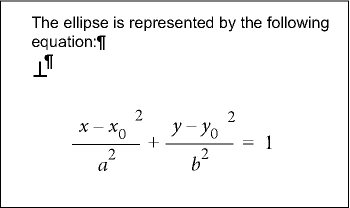6)Choose Shrink-Wrap Equation from the Equations pop-up menu. This option shrinks the frame around the equation and places the equation in the empty paragraph.

Shrink-wrapped equation in its own paragraph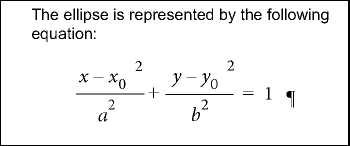#### Create an equation in a graphic frame with other objects

1)Click the Equations buttonat the right side of the document window.

2)Select a graphic frame or an object in a graphic frame.

3)On the Equations pod, choose one of the New Equation commands from the Equations pop-up menu. Small, Medium, and Large specify the font sizes used in the new equation.

A new equation object appears as a question mark inside the frame. The first math element you insert replaces the question mark prompt.

New equation object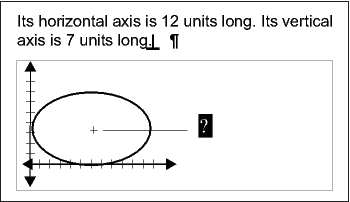4)Insert the math elements in the equation by typing the elements or by clicking items on the Equations pod.

5)To place the equation exactly where you want it, first select it by Control-clicking the equation.

6)Move the equation by doing one of the following:

Drag it with the mouse.

Move it with the arrow keys.

Position it precisely with the Graphics > Object Properties command.

September 30, 2016